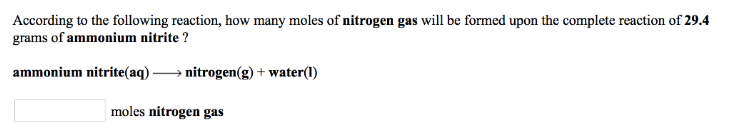# Problem: According to the following reaction, how many moles of nitrogen gas will be formed upon the complete reaction of 29.4 grams of ammonium nitrite?ammonium nitrite (aq) → nitrogen (g) + water (l)

###### FREE Expert Solution
84% (292 ratings)###### Problem Details

According to the following reaction, how many moles of nitrogen gas will be formed upon the complete reaction of 29.4 grams of ammonium nitrite?

ammonium nitrite (aq) → nitrogen (g) + water (l)